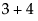You can often infer the meaning by the argument’s position. For example, the values 200 and 100 in size(200, 100) are implicit arguments. The first value is always interpreted as the width; the second value is always interpreted as the height. See also named argument.
The current data table is the data table that Current Data Table() either returns or is assigned.
The current row for scripting is defined to be zero (no row) by default. You can set a current row with Row() or For Each Row, and so forth.
An eliding operator is one that causes arguments on either side to combine and evaluate differently than if the statement were evaluated strictly left to right. For example, 12<a<13 is a range check to test whether a is between 12 and 13: JMP reads the whole expression before evaluating. If < did not elide, the expression would be evaluated left to right as (12<a)<13. In other words, it would check whether the result of the comparison (1 or 0, for false or true) is below 13, which of course would always yield 1 for true. The << operator (for object<<message, which is equivalent to Send(object, message) is another example of an eliding operator.
A function takes an argument or series of arguments inside parentheses after the function name. For example, the infix operator + has a function equivalent Add(). The statementsand Add(3, 4) are equivalent. All JSL’s operators have function equivalents, but not all functions have operator equivalents. For example, Sqrt(a) can be represented only by the function. Also see the Function operator for storing a function under a name.
In infix operator takes one argument on each side, such as + in arithmetic,, or the = in an assignment, a=7.
Something that can be the destination of an assignment. In this manual, L-value describes an expression that normally returns its current value but that can alternatively receive an assignment to set its value. For example, you would ordinarily use a function such as Row() to get the current row number and assign it to something else. For example, x=Row(). However, since Row is an L-value, you can also place it on the left side of an assignment to set its value. For example, Row()=10.
A list is a multiple-item data type entered in special brace { } notation or with the List operator. Lists enable scripts to work with many things at once, often in the place of a single thing.
A message is a JSL statement that is directed to an object, which knows how to execute the message.
A named argument is an optional argument that you select from a predetermined set and explicitly define. For example, title("My Histogram") in the Graph Box function is a named argument. In functions such as New Window, the title is not a named argument, because title is the first required argument.
Usually operator refers to a one- or two-character symbol such as + for addition or <= for less than or equal to.
A way to address a scriptable object in order to send it messages. For example,. column("age") or Current Data Table() or Bivariate. Typically a reference is stored in a global variable for convenience.
A scoping operator forces a name to be interpreted as a particular type of data element, for example the : operator in :name forces name to be resolved as a column; the :: operator in ::name forces name to be resolved as a global variable.

Help created on 9/19/2017